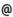## "Babes-Bolyai" University of Cluj-Napoca Faculty of Mathematics and Computer Science

 Numerical analysis
 Code Semes-ter Hours: C+S+L Credits Type Section MMN0002 4 2+2+2 7 compulsory Matematică MMN0002 4 2+2+2 6 compulsory Matematică informatică MMN0002 4 2+2+2 6 compulsory Matematici aplicate
 Teaching Staff in Charge
 Assoc.Prof. CHIOREAN Ioana Rodica, Ph.D., ioanacs.ubbcluj.ro
 Assoc.Prof. TRÎMBITAS Radu Tiberiu, Ph.D., traducs.ubbcluj.ro
 Asist. SOMOGYI Ildiko Mihaela, ilkovacsmath.ubbcluj.ro
 Lect. LISEI Hannelore-Inge, Ph.D., hannemath.ubbcluj.ro
 Aims Basic course in numerical analysis.
 Content 1. Error theory. Approximation. Types of errors. 2. Finite and divided differences. 3. Functions approximation. Interpolation. Uniform approximation. Linear and positive operators. 4. Approximation of linear functionals. Numerical derivatives. Numerical cuadratures. 5. Numerical solutions of some classes of operatorial equations. Direct and iterative methods for solving linear systems of equations. Multigrid methods. Numerical methods for solving nonlinear equations. Numerical methods for differential equations. 6. Example of solving practical problems from Physics, Biology, Chemistry by means of numerical analysis.
 References 1. COMAN,GH.: Analiza numerica, Ed. Libris, Cluj-Napoca, 1995. 2. STANCU,D.D.: Analiza numerica, curs si culegere de probleme, Univ. Babes-Bolyai Cluj-Napoca, 1977 (lito). 3. AGRATINI,OCTAVIAN- BLAGA,PETRU- CHIOREAN,IOANA- COMAN,GHEORGHE- STANCU,D.DIMITRIE- TRAMBITAS,RADU: Analiza numerica si teoria aproximarii(vol.I,II,III), Presa Univ.Clujeana, 2002 4. BLAGA,PETRU - COMAN,GHEORGHE, TRAMBITAS,RADU - VASARU,DANIELA- POP,SORIN: Analiza numerica, lucrari de laborator, Univ. Babes-Bolyai Cluj-Napoca, 1995 (lito). 5. GELFAND,A.O.: Calculul cu diferente finite, Ed. Tehnica Bucuresti 1956. 6. DEMIDOVICI,B.P.- MARON, A.: Elements de calcul numerique, Ed. Mir, Moscou, 1979.
 Assessment The final mark is the mean between the final written exam (60%) and periodical tests and individual work at the laborator classes (40%). For Computer Scientists the final score consists of: 2/5 written exam, 2/5 practical exam and 1/5 yearly activity.